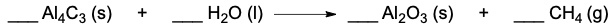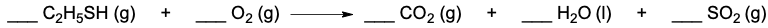## General Chemistry

Learn the toughest concepts covered in Chemistry with step-by-step video tutorials and practice problems by world-class tutors

3. Chemical Reactions

# Balancing Chemical Equations

Balancing Chemical Equations is the first step in future chemical calculations.

Balancing Chemical Equations
1
concept

## Balancing Chemical Equations1m
Play a video:
in this series of videos, we're gonna take a look at balancing chemical equations when balancing. Always make sure the type and number off Adams on both sides of the arrow are equal. We're going to say in a balanced equation. The numbers that are in red are referred to as the coefficients. So we have to one and two as the coefficients for this balanced equation, and we're talking about type and number of atoms. We'd say that these coefficients get distributed so this, too, would get distributed to this hydrogen. There's already two of them, so two times to give me four hydrogen. This is just the one, so one distributed is one times two, which is two oxygen's on the other side. The two gets distributed, so it be two times the two hydrogen would give me four hydrogen. But there's also one oxygen here, so two times one gives me to oxygen's, so the types of atoms are the same on both sides. I have hydrogen and hydrogen oxygen and oxygen, and then the numbers off each are equal on both sides. Four. Hydrogen, four hydrogen to oxygen's to oxygen's. This is how we ensure a chemical equation is balanced. The types and numbers of each atoms are the same on both sides of the arrow. Now click on the next video and let's take a look at an example question where we go more refined at our approach to balancing a chemical equation.
2
example

## Balancing Chemical Equations Example 15m
Play a video:
in this example question. It says White. The balanced equation for the following by inserting the correct coefficients in the blanks. Remember, those blanks are gonna hold our coefficients. Now, Step one. We set up a list for the elements that are reacting. It's and another list for the elements that are products. Now, remember that your reactant are the compounds or elements that come before the era, and then products are the ones that come after the arrow. If we take a look here, we make sure lists match up. We have carbon hydrogen oxygen as the elements on the react inside. Don't worry about the order of them on the product side. Just make sure that the list match up. Since I wrote Carbon first here, I have to write carbon first here and then h and then Oh, and so this has to follow suit h and then, oh, now let's count to see how many we have of them. So we're gonna go into step to start from the top and going down. Both lists determine how many of each element are present, so we can see here that there's a little sub script of four which mean there's four carbons. There's a subscript of 10 which you mean there's 10 hydrogen and a subscript of two wouldn't mean there's two oxygen's on the other side. Carbon. There's only one carbon here hydrogen. There's a sub script here of two, so there's two hydrogen oxygen. Make sure you look everywhere. On the product side, there's one oxygen here and also one oxygen here for a total of two. Oxygen's now starting for Step three. Starting from the top and going down, both list begin balancing each element to ensure they match Now. Sometimes you may have a decimal or a fraction as a coefficient, and so you must multiply the equation by two. So we're going to see if we have to do it in this case. So coming back up the list don't match. We know it's not balanced on the left side. My list is 4, 10 and 12 but on the product side it's 12 and two. So let's start out with the carbons. This is four, and this is only one, so I have to put a number here, a coefficient here that will multiply the number of carbons and make them match the number of carbons on the react Inside. The number at the place here is four, because that four gets distributed to the carbons. So four times one gives me four carbons now, but it also get distributed to the oxygen's four times. One oxygen is four oxygen's plus this one over here that's still around, so that'd be five. Carbons are the same, so that's good. Let's move down the hydrogen here. This is 10 but this is only two. So think of a number you can place here than when it multiplies. The two hydrogen is here gives me 10. The number would have to be five right, because five times to give me 10. But again, we have to think about what does this do to the oxygen as well, because the five is get getting distributed not only to the hydrogen but also to the number of oxygen's. So five times one is five. Oxygen's plus four mawr is nine oxygen's on this side, So now we have nine on this side, but only two on this side. So you have to think of a number that you can multiply two by to give you non so you can't multiply by four because that's only eight. You can't multiply by five because that would be 10. So in between would be 4545 times to gives me nine. But remember, we said in step full well, in step three, sometimes you have a decimal or a fraction as a coefficient. So you must multiply the equation by two. And we do this so that all the coefficients become whole numbers. So here, coming back up here, we're gonna multiply this whole thing times, too. When we multiply the entire equation by two, that, too gets distributed toe all of the coefficients, all of them get multiplied by two. So that means that our balance equation now becomes too. C four h 10 for gas plus nine for gas and then our products five times to gives me 10 h 20 as a liquid plus four times to gives me eight CEO, right. So that would those would be the coefficients from a balanced equation to 9, 10 and eight. So any time you're balancing a chemical equation, make your list and follow the steps necessary to balance
3
Problem

Write the balanced equation for the following by inserting the correct coefficients in the blanks.4
Problem

Determine the total sum of the coefficients after balancing the following equation.